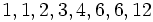Difference between revisions of "Landau's function"

Definition

Letbe a nonnegative integer. The Landau's function ofis defined in the following equivalent ways:

• The maximum possible value for the least common multiple of the parts in an unordered integer partition of.
• The maximum of the orders of all elements in the symmetric group of degree.

The value atis defined to be.

Behavior

The ID of the sequence in the Online Encyclopedia of Integer Sequences is A000793

=Initial values

The values of Landau's function foris.

Relation with other functions

• lcm of all numbers so far is the exponent of the symmetric group of degree.
• factorial is the order (i.e., cardinality) of the symmetric group of degree.